GeeksforGeeks App
Open AppBrowser
Continue

# ∈-NFA of Regular Language L = 0(0+1)*1 and L = (00)*1(11)*

Non-Deterministic Finite Automata and ∈-Non-Deterministic Finite Automata are almost the same except for their transition function and there are a few special rules for construction of ∈-NFA.

```∈-NFA is defined in 5 tuple representation {Q, q0, Σ, δ, F} where -
Q is the set of all states,
q0 is the initial state,
Σ is the set of input symbols,
δ is the transition function which is δ:Q × (Σ∪∈)->2Q and
F is the set of final states.```

Simple rules for construction of ∈-NFA :
∈-NFA for a+ :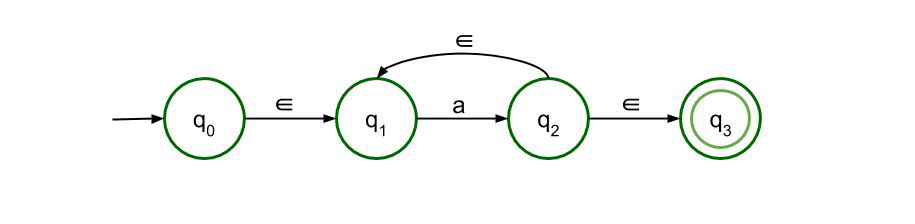This structure is for a+ which means there must be at least one ‘a’ in the expression. It is preceded by epsilon and also succeeded by one. There is epsilon feedback from state q2 to q1 so that there can be more than one ‘a’ in the expression.

∈-NFA for a* :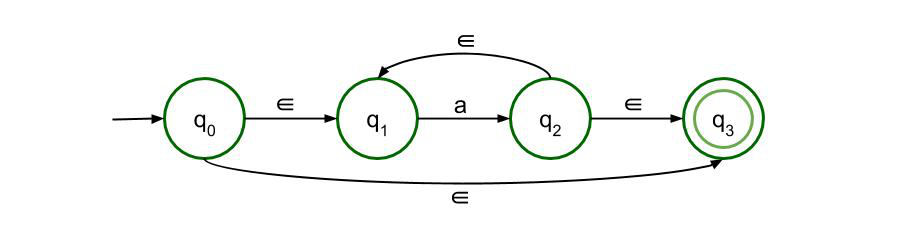This structure is for a* which means there can be any number of ‘a’ in the expression, even 0. The previous structure is just modified a bit so that even if there is no input symbol, i.e. if the input symbol is null, then also the expression is valid.

∈-NFA for a+b :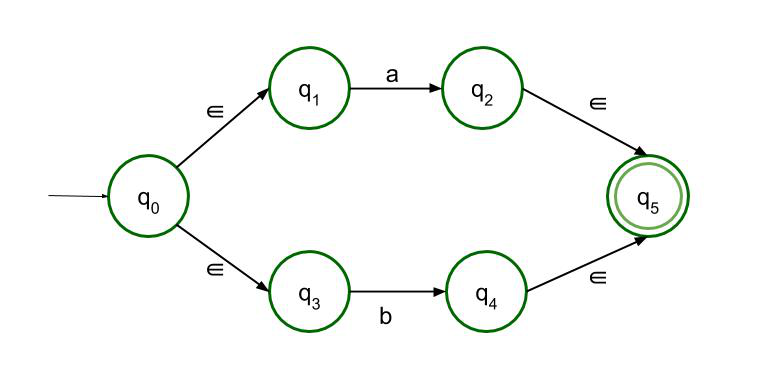This structure accepts either a or b as input. So there are two paths, both of which lead to the final state.

∈-NFA for ab :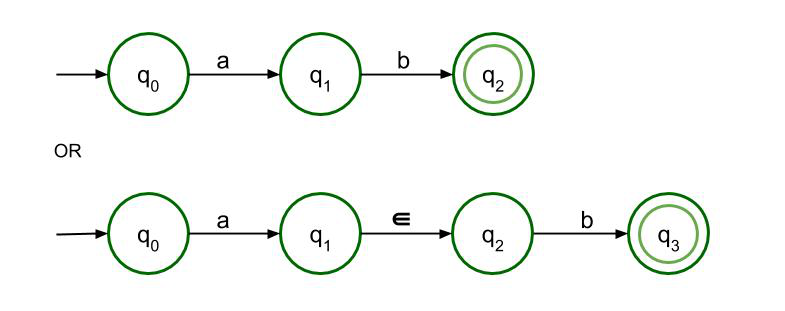For concatenation, a must be followed by b. Only then it can reach the final state. Both structures are allowed here but as it is ∈-NFA so the second structure is recommended.

∈-NFA for L = 0(0+1)*1 :

Following the above-mentioned rules, ∈-NFA of Regular Language L =0(0+1)*1 is to be constructed.

L = 0(0+1)*1 can be divided into three parts- 0, (0+1)*, 1. The second part, (0+1)*, will be drawn with the help of third rule (a+b) where a = 0 and b = 1, followed by second rule a* where a = (0+1). Below is its ∈-NFA.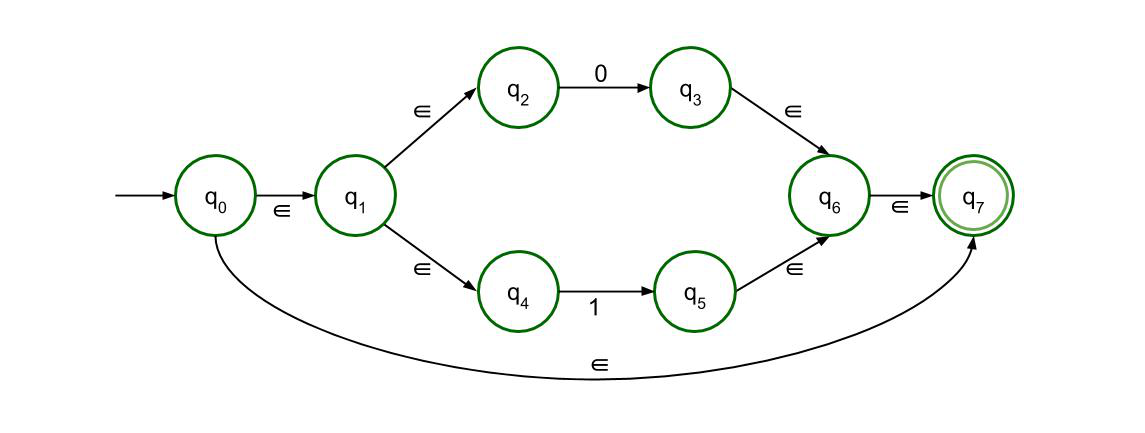Since 0 and 1 are just concatenated to the second part, the final ∈-NFA is drawn with the help of the fourth rule, ab.

The Final ∈-NFA will be –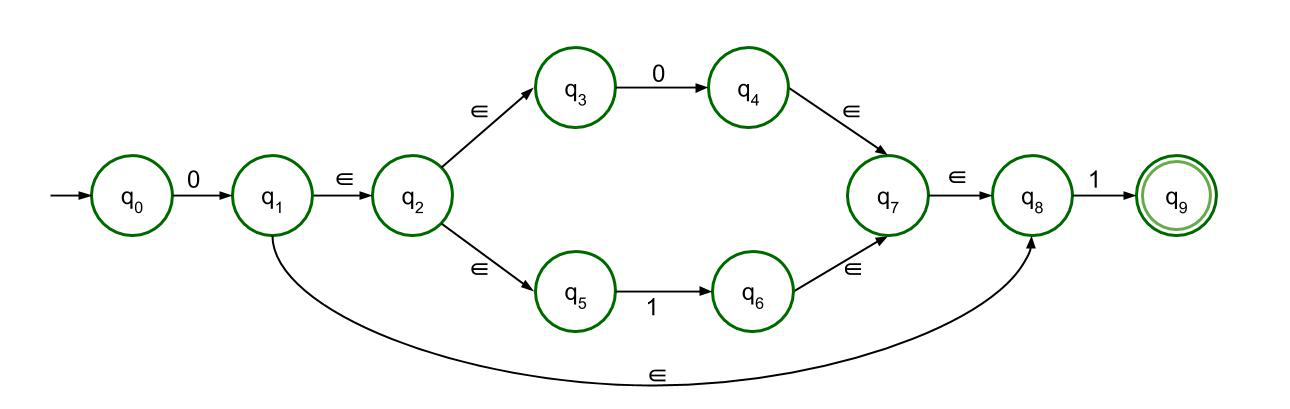∈-NFA for L = (00)*1(11)* :

Following the above-mentioned rules, ∈-NFA of Regular Language L = (00)*1(11)* is to be constructed.

L = (00)*.1.(11)* can be divided into three parts for the ease of constructing ∈-NFA. The first part is (00)*, the second part is 1 and the third one is (11)*. Since they are all concatenated to each other, the main structure is drawn with the help of the fourth rule, ab. Now, to understand the structure of the first part (00)*, the reference must be taken from the fourth, i.e. ab and the second rule, i.e. a*. First 00 are concatenated, then it is considered as one unit and applying the second rule, (00)* is drawn. Below is its ∈-NFA.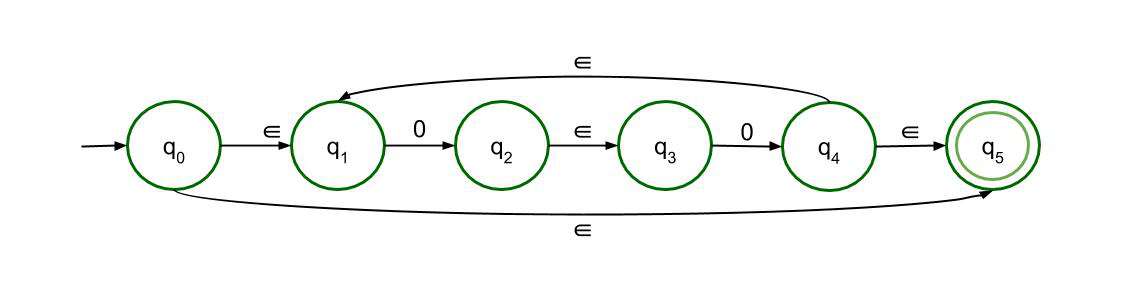Similarly, (11)* can be drawn. The second part of the language is easy. The first part and the third part are just concatenated with a ‘1’ in between.

The Final ∈-NFA will be –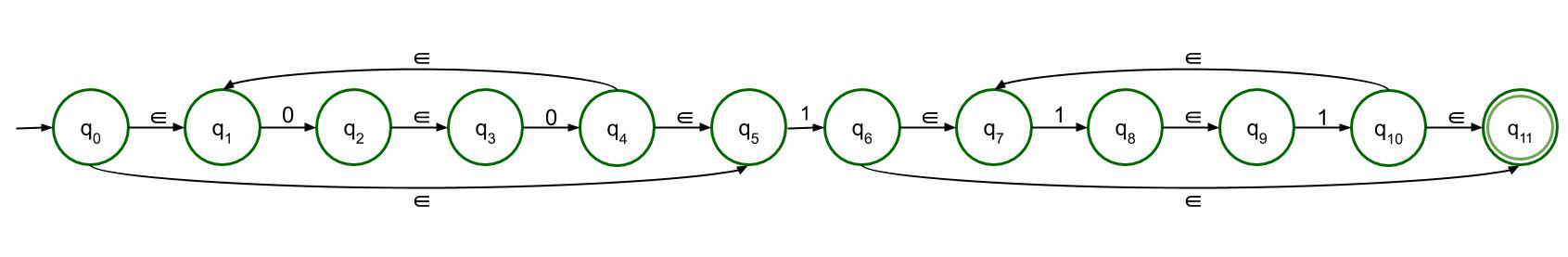My Personal Notes arrow_drop_up